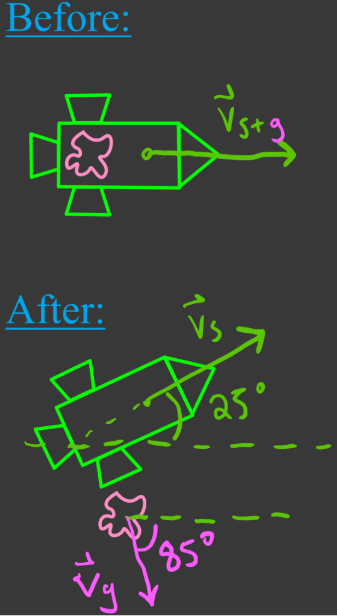# Conservation of momentum in two dimensions

### Conservation of momentum in two dimensions

#### Lessons

In this lesson, we will learn:

• Review of conservation of momentum
• Vector nature of momentum and conservation of momentum
• Problem solving with conservation of momentum in two dimensions

Notes:

• Momentum is a conserved quantity and a vector.
• In a collision between a set of objects, total momentum of the objects before collision = total momentum after collision.
• When using conservation of momentum on objects that move in two dimensions, use vector addition (tip-to-tail method).

Momentum

$\vec{p} = m \vec{v}:$ momentum, in kilogram meters per second (kg∙m/s)

$m:$ mass, in kilograms (kg)

$\vec{v}:$ velocity, in meters per second (m/s)

Impulse

$\vec{J} = \vec{F} \Delta t = \Delta \vec{p} = m\Delta \vec{v}$

$\vec{J}:$ impulse, in newton seconds (N∙s)

$\vec{F}:$ force, in newtons (N)

$\vec{t}:$ time, in seconds (s)

Conservation of Momentum

$\sum\vec{p}_i = \sum\vec{p}_f$

$\vec{p}_i:$ initial momentum, in kilogram meters per second (kg·m/s)

$\vec{p}_f:$ final momentum, in kilogram meters per second (kg·m/s)

Law of Sines

$\frac{a}{sinA} = \frac{b}{sinB} = \frac{c}{SinC}$
a,b,c: length of sides a,b,c
A,B,C: angles opposite sides a, b, c

Law of Cosines

$c^2 = a^2 + b^2 - 2ab \,cosC$

• Introduction

Introduction to conservation of momentum in two dimensions

• Review of conservation of momentum
• Vector nature of momentum and conservation of momentum

• 1.
$\bold{\sum\vec{p}_i = \sum\vec{p}_f}:$ Objects that bounce apart after collision in two dimensions
a)
1.25 kg ball A is moving at 3.40 m/s [E] when it strikes stationary 1.00 kg ball B. After the collision, ball A moves off at an angle of [60.0° N of E], and ball B moves off at an angle of [30.0° S of E]. Find the velocities of A and B after collision.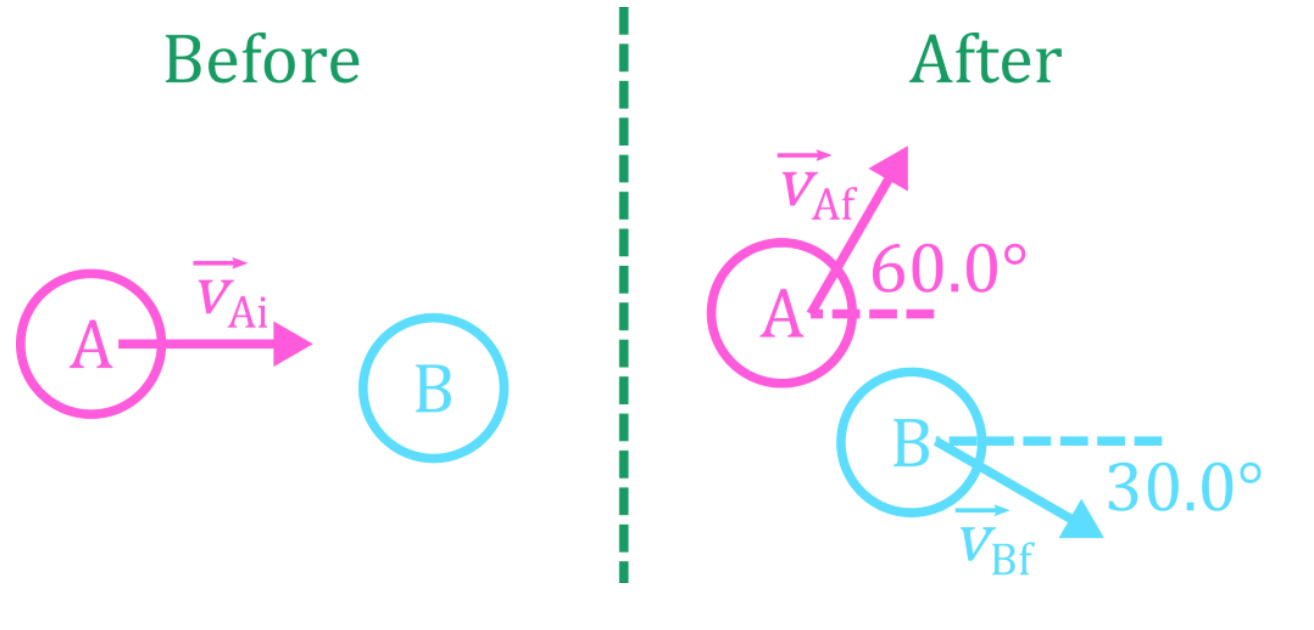b)
1.10 kg puck A travelling at 2.00 m/s [E] collides with 0.860 kg puck B moving 0.400 m/s [W]. After the collision, puck A moves off at 1.60 m/s [40.0° N of E]. Find the velocity of puck B.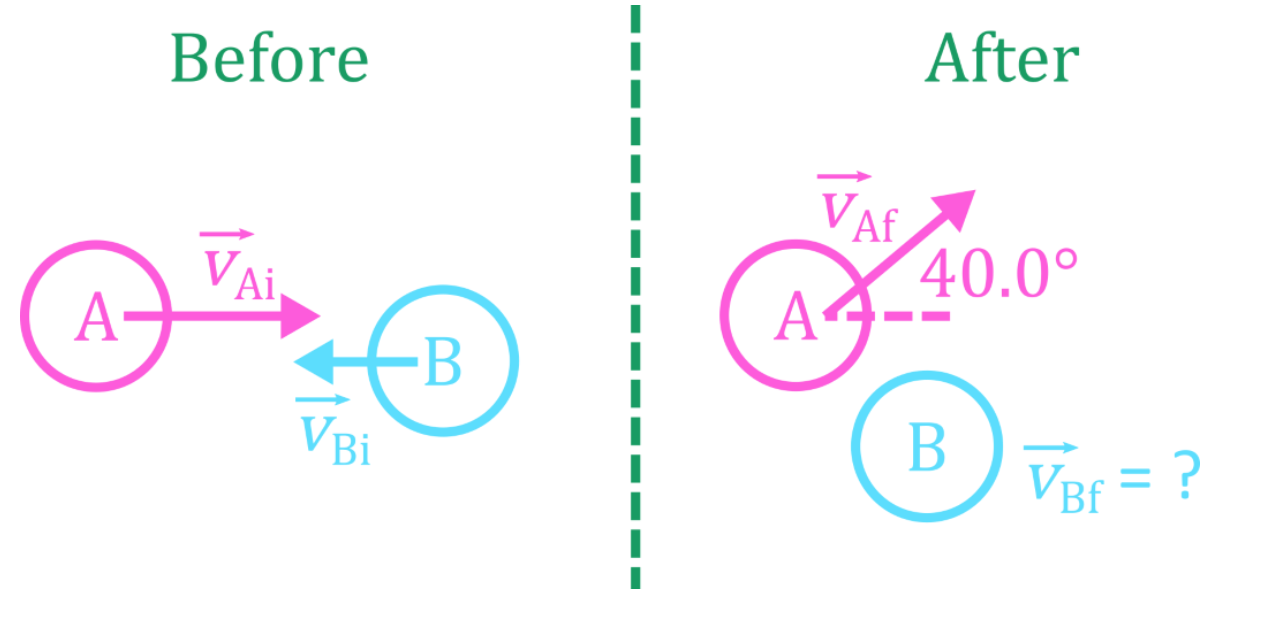• 2.
$\bold{\sum\vec{p}_i = \sum\vec{p}_f}:$ Objects that stick together after collision in two dimensions
a)
1100.0 kg car A travelling at 12.0 m/s [E] collides with 975 kg car B travelling 19.0 m/s [N]. The cars stick together. Find the velocity of the two vehicles after collision.

b)
0.50 kg magnetic puck A travelling at 1.1 m/s collides with 0.35 kg puck B travelling with an unknown velocity, as shown in the diagram. The two pucks stick together and travel due [E]. Find the initial velocity of the second puck.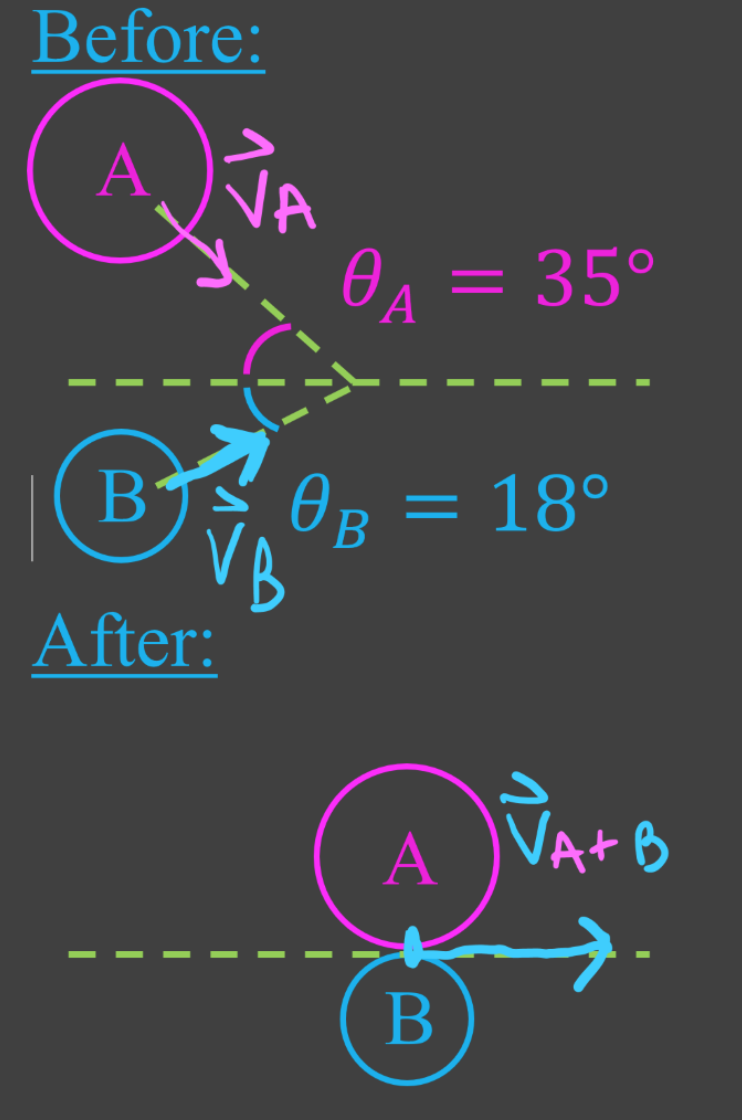• 3.
$\bold{\sum\vec{p}_i = \sum\vec{p}_f}:$ Objects that explode apart in two dimensions
a)
A bomb initially at rest explodes, sending three fragments in different directions as shown in the diagram. Find the velocity of the 0.71 kg fragment.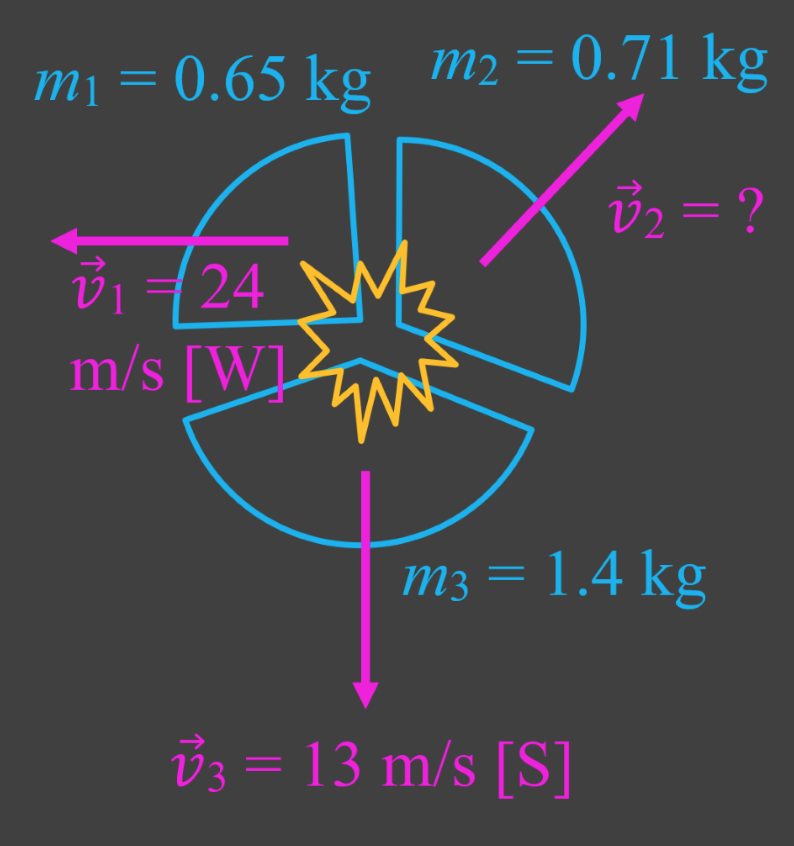b)

A 21.0 kg child holding a 0.755 kg snowball rides a 3.00 kg sled on a frictionless ice surface at 2.50 m/s [E]. The child throws the snowball, giving it an impulse due [N] relative to the sled. The snowball travels at 6.00 m/s immediately after it is thrown.

c)
Balls A, B, and C roll together as shown in the diagram, with all three balls colliding at the same time. Find the mass of ball C if the 3 balls are stationary after colliding.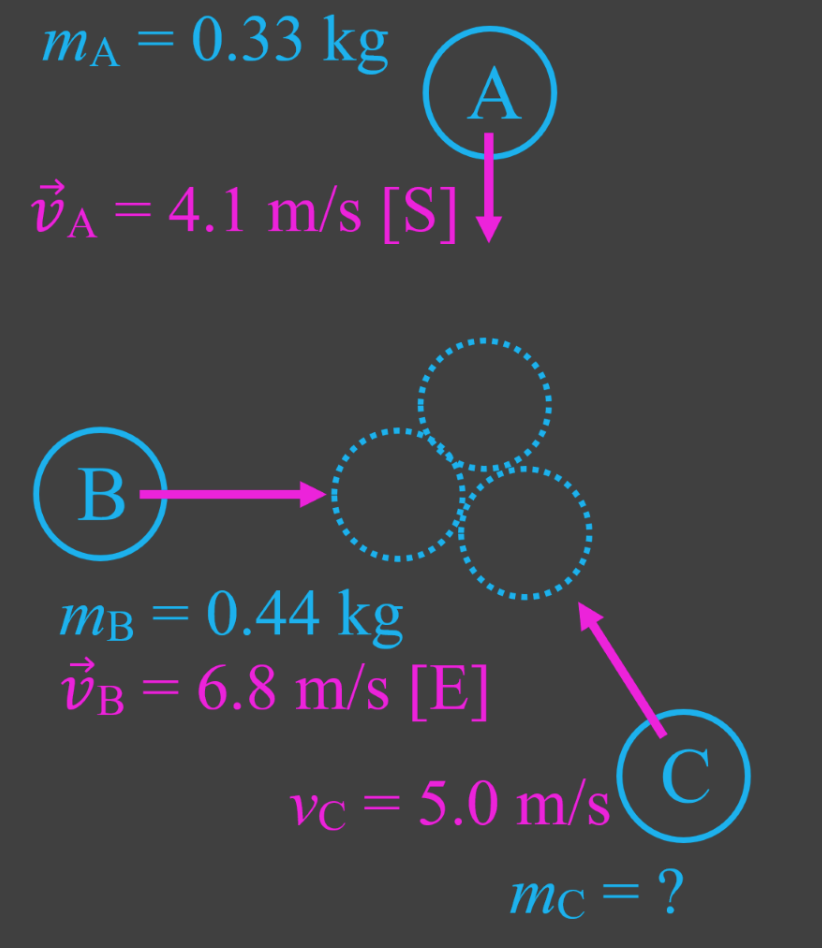d)
A spacecraft with a total mass of 8620 kg is travelling through space at a speed of 156 m/s. Control wants to adjust its direction by 25.0°, and increase its speed to 175 m/s. Gas is ejected though a thruster on the side of the craft, travelling at 85.0° clockwise from the craft's original direction of motion. Find the mass of gas ejected.